# Collisions Notes

## Collisions

• Collision between two particles is defined as mutual interaction between particles for a short interval of time as a result of which energy and momentum of particle changes.
• Collision between two billiard balls or between two automobiles on road are few examples of collisions from our everyday life. Even gas atoms and molecules at room temperature keep on colliding against each other.
• For the collision to take place , physical contact is not necessary. In cas of Rutherford alpha scattering experiment , the alpha particles are scattered due to electrostatic interaction between the alpha particles and the nucleus from a distance i.e., no physical contact occurs between the alpha particles and the nucleus.
• Thus , in physics collision is said to have occurred , if two particles physically collide with each other or even when the path of motion of one particle is affected by other.
• In the collision of two particles law of conservation of momentum always holds true but in some collisions Kinetic energy is not always conserved.
• Hence collisions are of two types on the basis of conservation of energy.
(i) Perfectly elastic collision
• Those collisions in which both momentum and kinetic energy of system are conserved are called elastic collisions for example elastic collision occurs between the molecules of a gas. This type of collision mostly takes place between the atoms, electrons and protons.
• Characteristics of elastic collision
(a) Total momentum is conserved.
(b) Total energy is conserved.
(c) Total kinetic energy is conserved.
(d) Total mechanical energy is not converted into any other form of energy.
(e) Forces involved during interaction are conservative in nature.
• Consider two particles whose masses are m1 and m2 respectively and they collide each other with velocity u1 and u2 and after collision their velocities become v1 and v2 respectively.
• If collision between these two particles is elastic one then from law of conservation of momentum we have
m1u1 + m2u2 = m1v1 + m2v2
and from the law of conservation of energy we have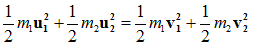(ii) Perfectly inelastic collision
• Those collisions in which momentum of system is conserved but kinetic energy of the system is not conserved are known as inelastic collision.
• Here in inelastic collision two bodies stick to each other after collision as a bullet hit its target and remain embedded in the target.
• In this case some of the kinetic energy is converted into heat or is used up in in doing work in deforming bodies for example when two cars collide their metal parts are bet out of shape.
• Characteristics of inelastic collision
(a) Total momentum is conserved.
(b) Total energy is conserved.
(c) Total kinetic energy is not conserved.
(d) A part or whole of whole mechanical energy may be converted into other forms of energy.
(e) Some or all forces involved during interaction are non-conservative in nature.
• Consider two particles whose masses are m1 and m2 respectively and they collide each other with velocity u1 and u2 respectively.
• If the collision between these two particles is inelastic then these two particles would stick to each other and after collision they move with velocity v then from law of conservation of momentum we have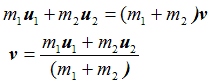• Kinetic energy of particles before collisions is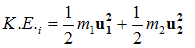and kinetic energy of particles after collisions is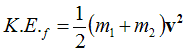by law of conservation of energy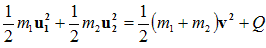where Q is the loss in kinetic energy of particles during collision.